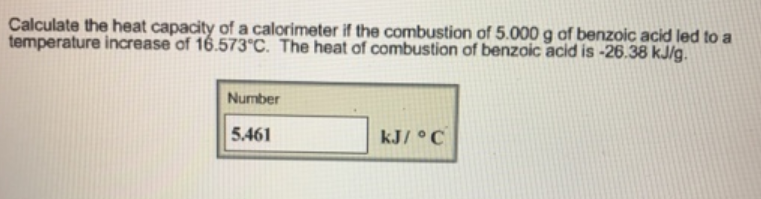# Problem: Calculate the heat capacity of a calorimeter if the combustion of 5.000 g of benzoic acid led to a temperature increase of 16.573°C. The heat of combustion of benzoic acid is -26.38 kJ/g.

###### FREE Expert Solution
82% (109 ratings)###### Problem Details

Calculate the heat capacity of a calorimeter if the combustion of 5.000 g of benzoic acid led to a temperature increase of 16.573°C. The heat of combustion of benzoic acid is -26.38 kJ/g.